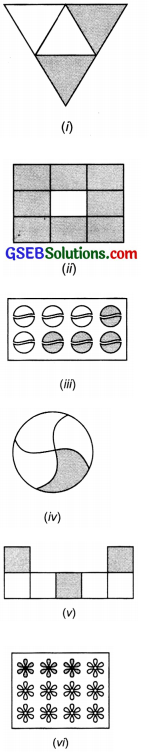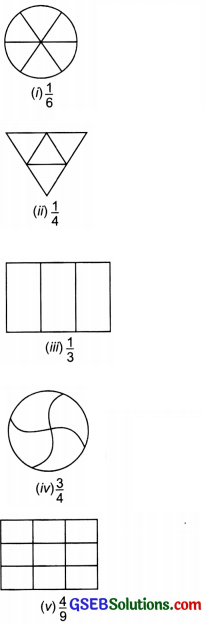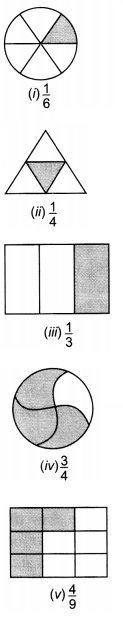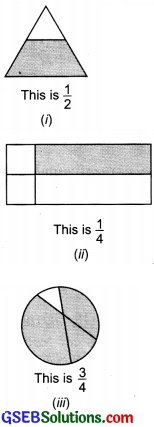# GSEB Solutions Class 6 Maths Chapter 7 Fractions Ex 7.1

Gujarat Board GSEB Textbook Solutions Class 6 Maths Chapter 7 Fractions Ex 7.1 Textbook Questions and Answers.

## Gujarat Board Textbook Solutions Class 6 Maths Chapter 7 Fractions Ex 7.1

Question 1.
Write the fraction representing the shaded portion.Solution:
(i) Total equal parts = 4
Fraction = $$\frac { 2 }{ 4 }$$

(ii) Total equal parts = 9
Fraction = $$\frac { 8 }{ 9 }$$

(iii) Number of total objects 8
Number of shaded objects = 4
Fraction = $$\frac { 4 }{ 8 }$$

(iv) Total equal parts = 4
Fraction = $$\frac { 1 }{ 4 }$$

(v) Total equal parts = 7
Fraction = $$\frac { 3 }{ 7 }$$

(vi) Number of total objects 12
Fraction = $$\frac { 3 }{ 12 }$$

(vii) Total number of objects 10
Number of shaded objects = 10
Fraction = $$\frac { 10 }{ 10 }$$

(viii) Total equal parts = 9
Fraction = $$\frac { 4 }{ 8 }$$

(ix) Total equal parts = 2
Fraction = $$\frac { 1 }{ 2 }$$

(x) Total equal parts = 2
Fraction = $$\frac { 1 }{ 2 }$$Question 2.
Colour the part according to the given fraction.Solution:Question 3.
Identify the error if anySolution:
(i) Since, the parts are not equal.
Shaded part is not $$\frac { 1 }{ 2 }$$

(ii) Since, the parts are not equal.
Shaded part is not a fraction $$\left(\frac{1}{4}\right)$$

(iii) Since, the parts are not equal.
Shaded part is not $$\frac { 3 }{ 4 }$$.Question 4.
What fraction of a day is 8 hours?
Solution:
Total number of hours in a day = 24
8 hours as a fraction = $$\frac { 8 }{ 24 }$$

Question 5.
What fraction of an hour is 40 minutes?
Solution:
1 hour = 60 minutes
40 minutes as a fraction = $$\frac { 40 }{ 60 }$$

Question 6.
Arva, Abhimanvu and Vivek shared lunch. Aya has brought two sandwiches, one made of vegetables and one of jam. The other two boys forgot to bring their lunch. Arya agreed to share his sandwiches so that each person will have an equal share of each sandwich.
(a) How can Arva divide his sandwiches so that – each person has an equal share?
(b) What part of a sandwich will each boy receive?
Solution:
(a) Arya will divide each sandwich into three equal parts. One part will be received by each of them.
(b) Each boy will get $$\frac { 1 }{ 3 }$$ parts or a fraction $$\frac { 1 }{ 3 }$$Question 7.
Kanchan dyes dresses. She has to dye 30 dresses. She has so Jar finished 20 dresses. What fraction of dresses has she finished?
Solution:
Number of dresses to be dyed = 30
Number of dresses finished = 20
Fraction of finished dresses = $$\frac { 20 }{ 30 }$$ = $$\frac { 2 }{ 3 }$$

Question 8.
Write the natural numbers from 2 to 12. What fraction of them are prime numbers?
Solution:
Numbers from 2 to 12 are: 2, 3, 4, 5, 6, 7, 8, 9, 10, 11, 12
Prime numbers are: 2, 3, 5, 7 and 11
Total given numbers = 11
Total number of prime numbers = 5
Required fraction = $$\frac { 5 }{ 11 }$$Question 9.
Write the natural numbers from 102 to 113. What fraction of them are prime numbers?
Solution:
Numbers from 102 to 113 are: 102, 103, 104, 105, 106, 107, 108. 109, 110, 111, 112, 113
Total number of given numbers = 12
Prime numbers are: 103, 107, 109, 113
Number of prime numbers = 4
Thus, the required fraction = $$\frac { 4 }{ 12 }$$

Question 10.
What fraction of these circles have x’s in them?Solution:
Total number of given circles = 8
Number of circles with X’s in them = 4
The required fraction = $$\frac { 4 }{ 8 }$$Question 11.
Kristin received a CD player for her birthday She bought 3 CDs and received 5 others as gifts. What fraction of her total CDs did she buy and what fraction did she receive as gifts?
Solution:
Number of CDs bought from market = 3
Fraction of CDs (purchased) = $$\frac { 3 }{ 8 }$$
Fraction of CDs (received as gifts) = $$\frac { 5 }{ 8 }$$